## ORIGINAL RESEARCH article

Front. Comput. Sci., 22 August 2022
Sec. Software
Volume 4 - 2022 | https://doi.org/10.3389/fcomp.2022.921454

# Convolutional kernel function algebraEdward Stow* andPaul H. J. Kelly
• Software Performance Optimisation Group, Department of Computing, Imperial College London, London, United Kingdom

Many systems for image manipulation, signal analysis, machine learning, and scientific computing make use of discrete convolutional filters that are known before computation begins. These contexts benefit from common sub-expression elimination to reduce the number of calculations required, both multiplications and additions. We present an algebra for describing convolutional kernels and filters at a sufficient level of abstraction to enable intuitive common sub-expression based optimizations through decomposing filters into smaller, repeated, kernels. This enables the creation of an enormous search space of potential implementations of filters via algebraic manipulation. We demonstrate how integral image and sliding window optimizations can be expressed in the context of common sub-expression elimination as well as show the direct use case for this algebra in massively SIMD multiply-free contexts such as in cellular processor arrays. We then show that this algebra is general enough to express and optimize kernels that use non-standard semi-rings to enable shortest path algorithms.

## 1. Introduction

Discrete convolutional kernels are a staple of vision and graphics processing from traditional edge detection algorithms to artificial intelligence and machine learning applications. As a subset of stencil functions they provide a way to describe transformations that are agnostic to absolute position within an image and instead the output at any pixel position is based only on a corresponding neighborhood of pixels in the input.

The aim of this algebra is to model the enormous space of semantically correct computational paths to produce specific kernels: decompositions. A decomposition is formed as an expression combining convolutional filters that produce the specific desired convolutional filter, where each of the filters in the decomposition models a hardware instruction or can otherwise be compiled for the target architecture. From this we can describe and compare methods of common sub-expression elimination (CSE) as well as other arithmetic reducing optimizations.

The algebra primarily formalizes the compilation search processes used in the AUKE (Debrunner et al., 2019) and Cain (Stow et al., 2022) compilers but we have found it enables us to model other stencil and convolution optimizations in very different contexts. AUKE and Cain are both compilers that target the SCAMP-5 Focal-Plane Sensor-Processor; this cellular processing array consists of a grid of 65,536 pixels each co-located with a very simple processing element (PE) (Dudek and Hicks, 2005). Every one of the PEs acts in lockstep completing the same instruction from a common bus making the SCAMP-5 a massively parallel SIMD architecture. With each PE having communication with its four local neighboring PEs, all in-plane image processing is done via local communication hops; furthermore each PE only has a very limited number of registers to store intermediate results and no addressable memory. The architecture is multiply-free as it has no multiply-unit; the only arithmetic operations available are addition, subtraction, negation, and division-by-2. As will be seen in Section 5, Cain's code generation process is directly linked to this algebra and serves as an open-source example of a search based compilation technique based on the symbolic manipulations that we have now formalized.

Within a larger compiler pipeline for convolutional filter optimizations on most architectures there will be several decisions and optimization passes outside of CSE that are not discussed within the context of this algebra such as data layout and locality optimizations, appropriate floating-point precision selection, kernel approximation, and quantization techniques, as well as loop unrolling and specific parallelization opportunities. The use for an algebra that provides a means to a search space of kernel decompositions is to help us make some of these decisions such as ones whereby the tolerance of an approximation can be reasoned about in terms of the arithmetic reduction it might enable. On the other hand, loop tiling and cache optimizations are generally applied after arithmetic reduction and are not modeled by the algebra. Hence the place for such an algebra in a convolutional kernel compiler pipeline for more traditional architectures lies early on in the stages at the point where a convolution has been concretely decided, perhaps based on some domain specific mathematics. We hope compiler creators can use this algebraic manipulation to search for decompositions that use a set of filters that can each be efficiently computed faster than a direct implementation of the original convolutional filter.

Our contributions include:

• An exploration of decomposition techniques for convolutional filters, targeted primarily at multiply-free, massively parallel devices such as Focal-Plane Sensor-Processors.

• A description of an algebra that provides the language and tools with which we can discuss arithmetic optimizations for constant-coefficient convolutional filters, as demonstrated through techniques such as kernel separability, sliding window optimizations, and summed-area tables.

• A formalization of the code-generation techniques deployed to various extents in the Cain and AUKE compilers for the SCAMP-5 FPSP.

• A demonstration that our algebra allows kernels using non-standard semi-rings to be optimized, as well as non-standard index spaces.

We begin with a brief look at the background followed by defining convolution kernels in the abstract, and the various operators and transformations that can be done with and to them. Next we look at how this theory is put into practice in tools like AUKE and Cain and show that we can use the algebra to describe how Devito (Luporini et al., 2020), a stencil optimization compiler targeting CPUs, manipulates convolutional kernel expressions and compare the extents to which CSE optimization is performed. This is followed by an exploration of further optimizations and concepts that can be modeled in the algebra. Next we discuss some of the related works and finally we discuss the future work for expanding the uses of this algebra and our conclusions on the proper place for this algebra within the world of convolutional filter optimizations.

## 2. Background

Optimizing various aspects of convolution filters, FIR filters, and stencils has a long history. Winograd showed that the lower bound on multiplicative complexity of general FIR filters is m+n−1 where m is the number of outputs given an input vector and an n-tap filter (Winograd, 1980). However, if the filter has constant coefficients the multiplicative complexity is a function of the weights themselves and so potentially much smaller, in extreme cases the lower bound becomes 0.

In constant coefficient multiply-free settings, using both digital and analog signals, the choice of representation for the coefficients can be used to increase the common-sub expressions within a filter allowing for reduced circuit complexity. The minimal signed digit (MSD) representation is a good example as every value can have multiple MSD representations (Park and Kang, 2001). This allows the choices of representations for each filter coefficient to be co-optimized to maximize reuse and minimize the required shifting/scaling and addition operations.

Separability of convolutions is a long established technique for reducing arithmetic redundancy in 2D filters (Lim, 1990) as well as in convolutional neural networks (CNNs) (Sifre, 2014). In CNNs however, it is generally used as a method to reduce the number of weights that need to be trained rather than to accelerate predefined filter weights. This is because in general an n-dimensional filter is not separable into n 1-dimensional filters. Separability takes advantage of the fact that a subset of convolutional kernels can be computed in two steps, each applying a simpler kernel with better performance characteristics such as fewer multiplies or a smaller effective size. Since this is often not possible, approximations are sometimes used, or those parts of the kernel that cannot be produced via separability are computed and added in another step.

There are several tools, languages, and compilers for preforming convolutional filter operations with high-performance and efficiency (Holewinski et al., 2012; Ragan-Kelley et al., 2013; Debrunner et al., 2019; Luporini et al., 2020; Stow et al., 2022). Cain and AUKE are both searching compilers in the sense that from a convolutional kernel they each construct a search graph of decomposition steps to find a execution path from input value to computed convolutional kernel in a massively SIMD multiply-free context. We show how an algebra for convolutional filters can enable a larger search space than could be constructed in some existing search based compilers thus giving the opportunity and possibility of finding more optimal execution paths.

## 3. Definition

The simple premise for convolutional operators is that for each coordinate i ∈ ℤd in an output array $\mathbf{\text{O}}\in {ℝ}^{{n}_{1}×{n}_{2}...×{n}_{d}}$, Oi is a linear combination of the neighbors of i from an input array A.

In the simple 2D case we can think of K as a matrix of weights that we overlay onto the input array at every position where it will fit. Then each weight is multiplied by the corresponding input and the sum over these is the output. K is a kernel, defined as a mapping from relative coordinates to the coefficients used in the linear combination.

In this definition however, the sizes of n1, n2, ...nd are ambiguous or we must assume infinite. This can become unintuitive for real world applications where the output needs to be stored in finite memory. To alleviate this we look to the function based definition of a convolution: the two inputs are functions and the output is a function on a relative shifts of the integral over the product of the functions: $\left(f*g\right)\left(t\right):={\int }_{-\infty }^{\infty }f\left(\tau \right)g\left(t-\tau \right)d\tau$. For discrete and bounded inputs we propose that we should consider both the input image and the convolutional kernel as partial functions—mappings from the index space to values, which will produce an output mapping. An index space, common between all these mappings, can be an n-dimensional integer vector as would be expected to index into an array or any other Abelian group: S = (V, ⊕). Examples of non-standard index spaces include discretizations of positions on a torus or rotations about a single axis. The values can be from any semi-ring though we shall use real numbers with ordinary addition and multiplication.

This definition mirrors the definition given in Equation 2 closely, but it also puts in place specific constraints on the domain of the output mapping such that the size of the output is unambiguous, and need not be infinite. This definition also means that the operation is not guaranteed to be commutative unless A and K have mappings for all values in their index spaces which would make them functions rather than partial-functions so that there are no indices where a result cannot be produced.

The most common index space are the 1 and 2 dimensional integer vector space with ordinary addition. This corresponds to 1D and 2D images and filters as we usually expect them and will be the focus of the notation and methods used. Extensions to regular convolutions such as dilated filters can be supported within our algebra, with dilated filters simply represented by replacing the kernel mappings with ones that reflect the dilated positions of coefficients. Strided convolutions are not so trivial as there is no standard convolution that can be used to represent the effect of a stride. A stride acts like a filter on the index space available but since this algebra deals with kernels defined in terms of relative offsets and a index space filter operation would need some origin to align the stride to we choose not to consider stride in kernels.

## 4. Formalization

In this section, we will describe the algebra and the manipulations that can be applied to kernels. Several pieces of notation to improve brevity are introduced throughout, for expanding the algebra from the simplest representations with addition to more complex decompositions into filters that model instruction and are carefully aware of multiple channels.

### 4.1. Notation

To represent the weights of convolutional kernels, matrices are often used; probably because they are easily readable for the common 1 and 2 dimension kernels. To extract the mapping K from a matrix we must decide on the origin and then we can simply read off the weights at each relative coordinate within the matrix.

Note that in this form we ignore zeros in the matrix as they will not contribute to the function. Unfortunately this leaves us with the ambiguity that matrix with zeros at the extremities is not fully accounted for in terms of the affect they will have on the size of the output array. For example:

This is pertinent because there are cases when it is more efficient to implement a “larger” but otherwise equal convolutional kernel or because the specific output size is of great importance.

Scaling to two dimensions is trivial for the matrix representation but for the wights mapping K becomes a function of a coordinate: K ∈ ℤ2 → ℝ, and writing out the mapping in set-notation becomes unwieldy for anything but the most trivial cases. To accurately represent a convolutional kernel with minimal ambiguity we propose the following notation: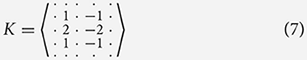In this representation, we enforce that there must always be an odd width and height so the center is unambiguous and we differentiate between zero and no value using a “·.” Unlike the matrix notation, therefore, this notation gives us the nice property that you can “zoom out” or add a ring of dots around the edge without any impact on the meaning. In the mapping notation, K from Equation (7) is defined as:

Addition of kernels is perhaps the most important operation, it is what allows for decomposition of more complex kernels into smaller manageable chunks, potentially reducing the overall complexity of producing a result. Quite simply:

The point is that the convolutional operator should always be distributive over operations on kernels:

Since the kernels and outputs are both weight mappings we can formalize addition for both as:

And we get the following properties of addition of kernels:

### 4.3. Scalar multiplication

It is trivial to multiply a kernel by a scalar, the process is simply to multiply each value in the mapping of a kernel by said scalar:

This gives us the following properties, where the × symbol is often removed since it is obvious in context:

With composition we will then see that multiplying by a scalar is equivalent to composing with a single entry kernel, with the scalar value in the center.

### 4.4. Composition

Next we can look at composing kernels, this provides us with a neat way to show repeated patterns in kernels and translate patterns and kernels using other, simple, kernels. We treat the composition of kernels much like the multiplication of numbers:

The exception is that there is not an inverse element for every kernel that has more than a single entry. Much like applying a convolution to an image, composing convolutions can be defined as:

This can be read as the repeated sum of copies of K weighted and translated by each mapping in J. Alternatively, we can define composition equivalently without relying on addition over kernels as an intermediary step:

Composition has a similar effect to convolution except that the requirement for the kernel to “fit” inside the input image is relaxed and the application of the kernel is inverted along each axis. Composition gives us a neat way to rewrite K from Equation (7):

We are able to decompose this kernel entirely, into a composition of factors, where each factor is a sum of single entry unit kernels (kernels with only one mapping whose value is 1). This decomposition suggests there are efficiencies to be had that are not obvious in the naive way to produce this kernel by simply multiplying inputs from different positions:

### 4.5. Channels

While the addition and composition of kernels goes a long way toward describing the decomposition of kernels, the definitions we have given do not account for kernels with multiple channels. These provide a means for a weight in a kernel to apply over a vector of inputs at the weight's relative position. We can motivate this with the example of RGB images, where we have three channels, or we might think about having a different channel for temperature, pressure, and each component of velocity etc. for weather simulation.

Naively, we could consider using vectors instead of scalar kernel coefficients such that A, K, OV ↦ ℝ|channels|, using element wise multiplication and addition. This, however, does not allow us to model the summation across the channels to produce a single scalar output. Alternatively, we could adjust V to include an extra dimension, this would allow us represent kernels that have multiple input channels and produce a single value, but then this kernel cannot be usefully decomposed as only the first kernel applied to the input could use multiple channels; the rest could only use the single channel produced by the first.

Instead we must produce a system where in kernels can act upon multiple inputs and produce multiple outputs. This allows us to reason about convolutional filters with multiple input channels as well as multiple outputs, each output being the result of a different kernel while exploiting common sub-expressions between these different kernels. For clarity we say that a kernel may only have one input channel and always produces a single output; and a filter can have multiple input and output channels.

For a set of Channels C:

In this definition the first C in the triple refers to the input channel and the last C refers to the output channel of a kernel coefficient. For simplicity of notation we assume that C can be labeled by the natural numbers. In our notation we use the idea of kernels that map from input to output to form filters, for example a filter that applies a vertical Sobel kernel to input channel 2 and outputs the result on channel 3 can be written as:

If we want to have multiple inputs channels combining to the same output then we use a vector notation as the coefficients in the filter: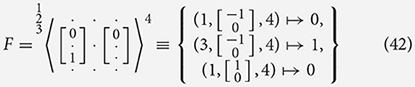To notate filters with multiple output channels we use a combination operator [F, G, ..., H], that accepts filters with only one distinct output channel each and produces a filter with multiple channels:

If the output channels are consecutive starting from 1 then we can omit the explicit channel naming.

We can then define composition on filters as:

In the example below we take the vertical Sobel filter and decompose it into effectively the same components as seen in Equation (38) but without using explicit addition. Instead we use a filter that performs an identical role while not requiring a larger set of rules in the algebra. From Equation (48) onward we omit the input and output channel names when they can be assumed from the filters themselves. In these places the names of the channels are not important as long as they match up.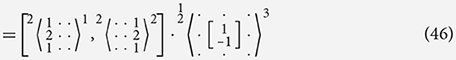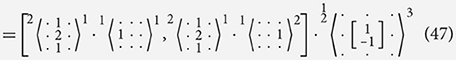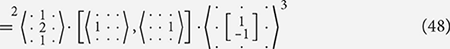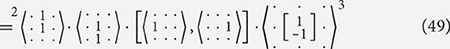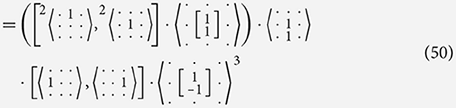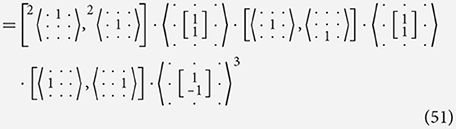In Equation (48), we see that distributively through filter combination holds with the general rules:

Associativity also holds but commutativity is not as straight forward with filter composition as with kernel composition. While it applies in the simple case, it does not always apply when more than one channel is used:

In modeling convolutions in architectures like SCAMP-5 it is helpful to represent each instruction as a filter acting on the PE registers as channels. It is useful to think about exactly what the semantics of an instruction used in this context would be. We have seen how we can use a filter that applies addition over multiple input channels but the meaning of an instruction generally has the nuance that it acts as a “pass-through” filter for all the channels that are not otherwise effected by the instruction. For example, an addition instruction over channels 1 and 2 that outputs its result into channel 3 also preserves the original values of 4, 5, 6, ...; and maybe 1 and 2 as well depending on implementation. We use the shorthand of an underscore to denote filters that we can assume have a “pass through”/identity kernel for all unspecified channels:

For example: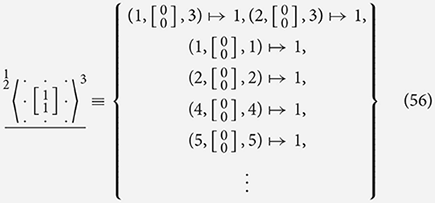We can use this concept to lower our Sobel filter decomposition further, removing the combination operator that would implies parallel execution of the simpler instructions: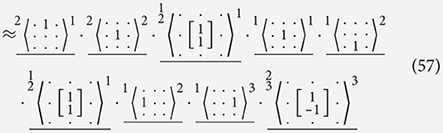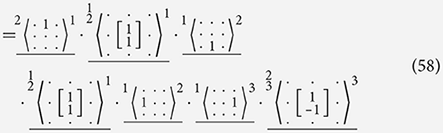In the final filter of Equation (57), we see that the choice of channels can not always be a direct copy of the pre-lowered decomposition as we must ensure that we do not overwrite results that are now sequentially needed. In Equation (58), we see that two identity kernels in our decomposition can be removed as these would effectively correspond to a noOp instruction. This lowering step changes the semantic meaning of the filter that we produce by introducing side effects on channels 1 and 2. The choice of channels that are effected can be made in anyway that satisfy the requirements and limitations on the channels and instructions.

### 4.6. Modeling implementation constraints

What we have seen so far is a nice mathematical representation of kernels and their operators, but there is an important aspect missing that ties the theory of our algebraic manipulations to the realities of computation, implementation constraints. In an implementation we must be aware of the storage and memory requirements of the platform performing each kernel operation. This means, for example, that to store an image we need to hold each pixel value in some form of memory.

In traditional CPUs this amounts to understanding the specific sizes of arrays that might need to store intermediate results. For more specialized and novel processors like Focal-plane Sensor-processors we must consider which processors in an array of processing elements must be active to produce a result of a size we want.

We propose that every operation required to produce a convolutional filter can be expressed as a filter—and so can be written in our kernel algebra. Addition and multiplication are trivial and we have already seen them both. In a CPU we see that when iterating through an array to compute a kernel, accessing elements of the input at relative positions is captured by a unit single entry kernel as seen in Equation (39).

A fundamental constraint in most processor architectures is that the result of applying a kernel is a value that must be stored somewhere. The affect on the kernels that can be computed is simple:

A kernel can only be applied where memory exists to store the result.

In a CPU context this means there must be an output array large enough to store the value, and in an FPSP like SCAMP-5 it means the result is stored in a PE at the center of the kernel.

Since we are now dealing with instructions encoded as filters, the order in which we apply the instructions becomes important, so we define a new left-associative operator “⊳” to represent composing two kernels and then adding a another kernel that encodes the constraints in the architecture. ⊳ is not commutative or associative since instructions are processed in order. Starting with I·, the identity filter under composition, we apply a “Move Down” instruction, that models the SCAMP-5 architecture, encoded in the filter.

For each + in the instruction we add a corresponding 0 to the result of the composition. In this case it encodes our principal that the output must be stored at the center of the kernel. This does not mean the result is stored back into the input array but that the output array has a place for the value to go. The meaning is mostly lost on CPUs where the there are few constraints on what memory can be accessed relative to current position of a kernel as we scan across the input. However, when composing multiple modeled SCAMP-5 instructions we see how this affects the shape of the kernels actually being calculated:

We can express an entire program such that working through the compositions will result in true size of the program being expressed. The first step is to take our decomposition, ensuring that all the filters it uses express available instructions in our instruction-set. To improve clarity and demonstrate the direct mapping to SIMD CPA instructions we can simply define an add(a, b, c) (2 operand) instruction in place of writing out kernels:

From Equation (58), we see that all the filters are simple translations that we will assume are in our instruction set, or 2 operand additions and subtractions.

We can now work through this program step by step—in execution order.

This all means that the example kernel in Equation (7) hasn't been perfectly compiled into instructions but instead we have modeled both the side effects and corrected filter shape given an implementation that allows us to understand the real consequences of the operation while performing optimizations on the way.

## 5. Modeling existing works with our Kernel algebra

Both the AUKE compiler (Debrunner et al., 2019) and Cain compiler (Stow et al., 2022) accept a kernel that is first approximated such that the coefficients are integers multiples of binary fractions, $\frac{n}{{2}^{d}}$, they then work backwards in their own ways to produce a plan for computing the kernel. In AUKE a kernel is represented by “atoms”—indivisible units at various positions within the kernel each worth $\frac{1}{{2}^{d}}$ that make up the kernel coefficients. This kernel of atoms is decomposed by splitting it into parts that can be shifted and combined. This is done to produce 1, 2, or 3 simpler kernels labeled U, L, and R that can be combined to output the desired kernel F. This is repeated until we have a single identity kernel, which will have 2d atoms in the center. From these transformations a data flow graph can be built. Modeling the kernels as a collection of atoms is the quantified basis that our algebra builds on and formalizes. Hence Debrunner's method is captured by our notion of decomposition fairly simply: each of these transformations in AUKE has the following form: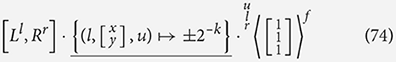where x and y allow for shifting of L to produce U, and $±{2}^{-k},k\in {ℤ}_{0}^{+}$ allows for negation and repeated dividing by two AUKE uses these transformations to produce a graph of kernel states that can then be manipulated in an equivalent fashion to the algebraic manipulations we use in decomposing kernels. These are then trivially reduced to individual SCAMP-5 instructions for shifting, adding, subtracting, and dividing.

In Cain kernels are represented as integer quantities of atoms, an optimization on the approach in AUKE. Cain has a similar but different approach to creating a searching where each available instruction in SCAMP-5 is directly represented as a filter as seen for decompositions in Section 4.6. Cain finds ways to apply the inverse of these instructions to the input filter to produce a list of many potential filters that by the application of that one instruction will produce the desired resulting filter. Again, this process is repeated until the filter to compile is simply the identity filter. This method produces a list of instructions that are both directly the instructions to apply register allocation to, and each a filter as can be represented in our algebra. Instructions like addition are not simply invert-able so every possible pair of filters that would sum to the desired result must be included in the search graph for an exhaustive search.

For a given set of kernels F = {K1, ..., Kn} where Kx ∈ (ℤ × ℤ)↦ℤ and using simple add, subtract, and neighbor-move instructions, Cain's search graph can be produced as:

If choices for L and R are exhaustive for all instructions then we can say that the search space includes every way to achieve the desired result given the available instructions and so includes the optimal solution. An exhaustive search is intractable because there are an infinite number of ways to produce any filter as the addition of two filters, but through heuristics Cain is able to ignore unlikely candidates and steer the search toward effective and profitable optimizations.

Devito is a python based framework that automates much of the process of numerically solving partial-differential equations using the finite-difference method (Luporini et al., 2020). The framework heavily relies on producing high-performance C++ code that compute stencils to solve problems such as full waveform inversion. Within Devito, stencil optimizations include symbolic analysis that finds common sub-expressions between coefficients at different positions in a stencil. Their optimizations are described in Section 5.1 of Luporini et al. (2020) and here we show how these can map into our algebra, starting with reused variables as coefficients:

Factorizations of the stencil can be found where the coefficients are equal:

This factorization is made as a distinct optimization to extraction in which Devito explicitly creates a temporary array to store an intermediary result. This difference is a choice of where within a series of nested loops the computation should take place. Such a choice can be clearly represented with bracketing to explicitly show the order of execution though composition does not formally define this schedule—this would be for a lowering step that interprets this expression as presented to control:

Lastly, Devito extracts “shift invariant” expressions. In Devito, this is a separate analysis task to factorization and extraction but in this algebra they are clearly related and can be transformed between one another:

Devito goes on to perform several optimizations at the iteration-space and loop levels to reduce data movement and maximize cache hit-rate to improve performance. It is a limitation of this algebra that such optimizations are not obviously representable. Optimizations that improve spacial and temporal locality based on the assumption of loops in a CPU architecture rather than reducing the total number of operations required are step beyond the focus of this work.

We have shown here that this algebra can be used to produce an enormous search space of decompositions that encompasses those used in exiting tools for arithmetic reduction.

## 6. Further uses

In this section, we describe optimizations and generalizations of the algebra that go beyond the standard methods of common sub-expression elimination. These are well-known techniques that on first glance appear to be separate from the focus of the algebra but we show how they can be modeled in using our existing rules, with no or minimal caveats and qualifications.

### 6.1. Recursion

A well known optimization for computing a 1D box filter kernel of width n is to consider the kernel as a sliding window with an accumulation variable. Every time we slide the window across one space to compute the kernel output at the new position we simply take the previous value, subtract the value that has just been left out of the window, and add the value that has just entered the window. This means for no matter how large n is, each new output only takes one subtraction and one addition.

If n = 5 then we have as our kernel:

From here we can represent this optimization quite simply:

We can clearly see the optimization as described: taking the previous value, subtracting the element as it leaves the window and adding the new element in. If we tried to produce instructions for this kernel naively we would have an infinitely long list of instruction as this expression does not tell us how to produce the base case. But if we were to produce a base case without recursion this decomposition shows that as long as we iterate to the right (such that the K to our left already exists) then we can make this optimization. While the example shown is a simple box filter kernel it is clear that the same technique can be applied to any arbitrary filter, with mixed results in terms of potential performance improvement.

### 6.2. Integral image

To describe Integral Maps, also called Summed-area Tables, we first need to represent infinite kernels: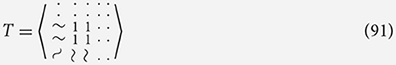Here we see that this kernel could only be used on a theoretical infinite input array. But like with the recursive case if we assume a base case or boundary then this kernel is simply a representation of producing an integral image or Summed-area table. We can then use this kernel like any other to produce an output such a box filter in the classic use case: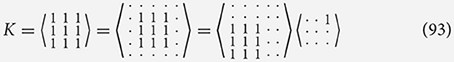This shows how we can represent the efficient decomposition of a simple Box filter assuming we have already calculated T.

### 6.3. Non-standard semi-rings

Using linear algebra paradigms and semi-rings allows us to describe graph algorithms such as shortest path algorithms where by the standard multiplication and addition operators are replaced with addition and minimum operations. A row vector of nodes repeatedly multiplied by an adjacency weight matrix will produce a vector of shortest distances:

In a similar way, we can look at how non-standard semi-rings can be used in convolutions, for example the min-sum semi-ring could be used to find the number of steps it would take a knight to move to various point on a chess board. In this case A is the board with its size (8, 8) but that conceptually at all other indices of A, not shown, we have ∞ which means we can assume the size of A*K is not any smaller.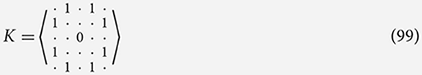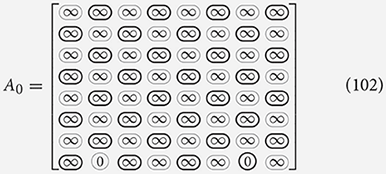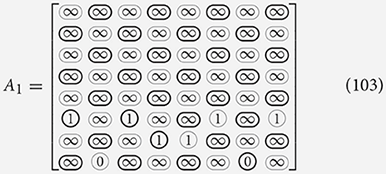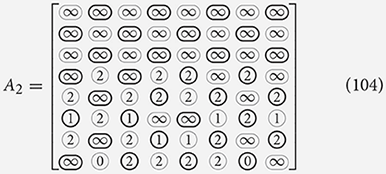Like with the linear algebra example, through repeated application we can achieve a map of shortest path lengths to have one of our knights reach the a target. We can still perform decompositions on K but we must be careful to obey the new meanings of multiplication and addition: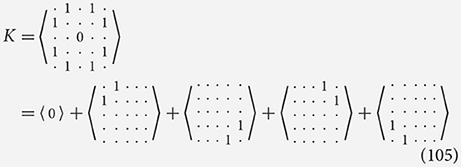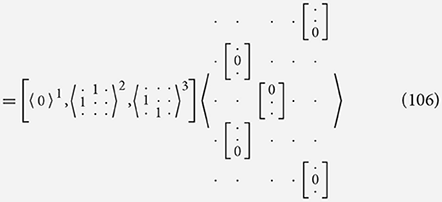## 7. Related work

General background in covered in Section 2; in this section we focus on prior work in compilers for common sub-expression elimination and symbolic expressions of convolutional filters used to automate optimization decisions, and how this relates to this work. There are several works that attempt to reduce the arithmetic complexity of constant coefficient convolutional filters. We have already discussed Cain and AUKE, two compilers for multiply-free convolutional filters; as well as Devito's Symbolic Engine, a tool for exploiting common sub-expressions in stencils produced in the implementation of the finite-difference method, in Section 5.

Domain specific programming languages such as Halide improve the maintainability and performance of convolutional filter code (Ragan-Kelley et al., 2013). Halide decouples the functionality of chains of filters from the execution policy. This means algorithmic development and performance optimization can be tackled separately. Users specify filters in a functional language embedded in C++ and can then either define a schedule of execution manually or use an automated scheduler. While Halide is able to transform a functional filter specifications with constant coefficients to high performance executables it does not perform symbolic analysis of the filters to determine automatically if the filter is separable or could be factorized. We see this as an opportunity for a producing a tool that pipelines filters specified in our algebra into Halide. While our algebra aims to tackle arithmetic reduction and common sub-expression elimination it is clear that there is a great gap between flop-optimal filter design and executable functions that Halide could provide.

In Stock et al. (2014), the associativity and commutativity of kernel weights are exploited to enable better reuse of input data. In a naive optimization of an n×n kernel we might use a loop nest over the rows and columns of the data, with unrolled loops over the kernel to perform the product and summation into an output array. For maximum reuse between consecutive iterations along the columns we need n×(n−1) registers, this means only n new values each iteration need to be loaded from the cache. The problem is that this causes register spilling for higher values of n which degrades the performance. Stock et al. developed strategies reduce the number of registers required. While this work utilizes associativity and commutativity to reduce register pressure and increase data reuse it is independent of common sub-expressions within the kernel weights and so like Halide, it is distinct from our algebra.

Linnea is a linear algebra compiler that works from an abstract syntax tree to encoding an expression to search for high-performance ways to compute said expression (Barthels et al., 2021). Linnea creates a directed acyclic graph, the root node being the whole expression, and each edge being a step that would simplify the parent node—either by computing some value or by symbolically rewriting the expression. By using knowledge of liner algebra in the form of lemmas and inference rules, Linnea can lower the flop-count of algorithms for calculating input expressions. The approach taken by Linnea appeals as a potential future work for our algebra; now we have a formalized set of rules for rewriting and inferring properties that can be applied to expressions of convolutional kernels.

In existing works on common sub-expression elimination, the process is often described and notated using an array index notation or wiring diagrams (Pasko et al., 1999; Mori et al., 2012; Fukushima et al., 2018). While these notations are sufficient for explanation, they do not make the limitations of the manipulations being used apparent, and so make it difficult to consider how different optimizations could be combined. It is clear that the varied methods for communicating how convolutional kernel optimizations are performed are not always compatible, this can mean readers must gain an intuition for the optimization before find it is equivalent or related to another method, as well as being able to decide correctness.

## 8. Future work

The algebra we present is designed with compilers in mind, it enables us to produce a more formal understanding of the transformations that would reduce arithmetic cost in executing convolutions. For this to be useful and practical in implementation we must consider how a cost function can be applied to arbitrary expressions in our algebra. The Cain compiler uses a cost model partly based on picking which child node reduces the number of non-zeros in the kernels to produce while maximizing common patterns between kernels. This model has been effective on the SCAMP-5 FPSP but it relies on the premise that each edge in the search graph is one instruction on the device that each take the same amount of time: there are no control flows, cache structures, or out of register storage of intermediate results to consider. Future work would include developing a framework for modeling the cost of different expressions in our algebra for more traditional CPU architectures, FPGAs, and GPUs.

One route to a generalized cost model for the algebra might include augmenting the algebra to explicitly provide context in terms of iteration spaces, storage of intermediaries, and tiling information. While arithmetic complexity of decompositions can be easily derived from expressions in the algebra it is well-known that this is often not the bottleneck and reducing accesses to main memory is more important, sometimes even at the expense of recomputing values. It is clear that some decompositions expose ways to combine effectively one-dimensional kernels in more complex ways than traditional separability to produce higher dimensional filters and so provide great opportunities for parallelism and vectorization in the production of intermediate results. These sorts of insights for specific kernels could be automated and exploited if a cost model were able to predict the further optimizability of kernels that takes into account locality and parallelism.

With an effective cost model it would become a feasible endeavor to implement a general searching compiler. The search space for decompositions is enormous, even when the possible steps of decomposition are severely restricted as in AUKE and Cain the search can only be done exhaustively for very small examples. In Cain's model the cost function of a whole program is very reliably found as just the total number of kernels in the decomposition, but this is not an viable strategy in general.

## 9. Conclusion

The space for optimizing convolutional filters is large and diverse. We have presented an algebra that can be used as a language to describe and analyse several types of arithmetic reduction optimization used for different architectures, such as separability on SCAMP-5 and sliding window optimizations that are irrelevant on SCAMP-5 but are common place in CPU convolution algorithms. This algebra underpins the optimizations and code generation used in the Cain compiler and for various processor architectures it provides a meaningful abstraction for understanding and finding high-level optimizations. The algebra is limited in that it does not intuitively consider the stride of kernels, neither does it allow us to reason about tiling the convolution for cache efficiency. While these are important features and optimizations, we claim that this algebra provides a more abstract view of the problem and in no way claim this is the only step in the optimization path.

Our convolutional kernel algebra sufficiently describes many abstract optimizations used for stencil filters, FIR filters, and convolutional filters; and we expect there are more that we have not shown that could be found given an extensive search of the problem space as defined by the algebra. We show simple examples of sliding window and summed-area-table optimizations but the same techniques can be applied to many different kernels given the tools to manipulate the kernels safely. Since this system allows kernels to be decomposed into other kernels, its principals could be used without significant changes to the overall compilation-pipeline of many existing frameworks.

## Data availability statement

The original contributions presented in the study are included in the article/supplementary material, further inquiries can be directed to the corresponding author.

## Author contributions

Work conducted by ES under the supervision and guidance of PK. All authors contributed to the article and approved the submitted version.

## Funding

This study received funding from the EPSRC (EP/W007789/1 and EP/R029423/1) and Dyson Technology Limited. The funders were not involved in the study design, collection, analysis, interpretation of data, the writing of this article, and the decision to submit it for publication.

## Conflict of interest

The authors declare that the research was conducted in the absence of any commercial or financial relationships that could be construed as a potential conflict of interest.

## Publisher's note

All claims expressed in this article are solely those of the authors and do not necessarily represent those of their affiliated organizations, or those of the publisher, the editors and the reviewers. Any product that may be evaluated in this article, or claim that may be made by its manufacturer, is not guaranteed or endorsed by the publisher.

## References

Barthels, H., Psarras, C., and Bientinesi, P. (2021). Linnea: automatic generation of efficient linear algebra programs. ACM Trans. Math. Softw. 47, 1–26. doi: 10.48550/arXiv.1912.12924

Debrunner, T., Saeedi, S., and Kelly, P. H. J. (2019). AUKE: automatic kernel code generation for an Analogue SIMD Focal-Plane Sensor-Processor Array. ACM Trans. Archit. Code Optim. 15, 1–26. doi: 10.1145/3291055

Dudek, P., and Hicks, P. (2005). A general-purpose processor-per-pixel analog simd vision chip. IEEE Trans. Circuits Syst. I Regul. Pap. 52, 13–20. doi: 10.1109/TCSI.2004.840093

Fukushima, N., Maeda, Y., Kawasaki, Y., Nakamura, M., Tsumura, T., Sugimoto, K., et al. (2018). “Efficient computational scheduling of box and gaussian fir filtering for cpu microarchitecture,” in 2018 Asia-Pacific Signal and Information Processing Association Annual Summit and Conference (APSIPA ASC) (Honolulu, HI), 875–879.

Holewinski, J., Pouchet, L.-N., and Sadayappan, P. (2012). “High-performance code generation for stencil computations on gpu architectures,” in Proceedings of the 26th ACM International Conference on Supercomputing, ICS '12 (New York, NY: Association for Computing Machinery), 311–320.

Lim, J. S. (1990). Two-Dimensional Signal and Image Processing. Prentice Hall Signal Processing Series. London: Prentice-Hall International.

Luporini, F., Louboutin, M., Lange, M., Kukreja, N., Witte, P., Hückelheim, J., et al. (2020). Architecture and performance of devito, a system for automated stencil computation. ACM Trans. Math. Softw. 46, 1–28. doi: 10.1145/3374916

Mori, J. Y., Llanos, C. H., and Berger, P. A. (2012). “Kernel analysis for architecture design trade off in convolution-based image filtering,” in 2012 25th Symposium on Integrated Circuits and Systems Design (SBCCI) (Brasilia), 1–6.

Park, I.-C., and Kang, H.-J. (2001). “Digital filter synthesis based on minimal signed digit representation,” in Proceedings of the 38th Design Automation Conference (IEEE Cat. No.01CH37232) (Las Vegas, NV), 468–473.

Pasko, R., Schaumont, P., Derudder, V., Vernalde, S., and Durackova, D. (1999). A new algorithm for elimination of common subexpressions. IEEE Trans. Comput. Aided Des. Integr. Circuits Syst. 18, 58–68. doi: 10.1109/ASAP.2000.862402

Ragan-Kelley, J., Barnes, C., Adams, A., Paris, S., Durand, F., and Amarasinghe, S. (2013). Halide: A language and compiler for optimizing parallelism, locality, and recomputation in image processing pipelines. SIGPLAN Not. 48, 519–530. doi: 10.1145/2499370.2462176

Sifre, L. (2014). Rigid-motion scattering for image classification (Ph.D. thesis). Ecole Polytechnique, Palaiseau, France.

Stock, K., Kong, M., Grosser, T., Pouchet, L.-N., Rastello, F., Ramanujam, J., et al. (2014). A framework for enhancing data reuse via associative reordering. SIGPLAN Not. 49, 65–76. doi: 10.1145/2594291.2594342

Stow, E., Murai, R., Saeedi, S., and Kelly, P. H. J. (2022). “Cain: automatic code generation for simultaneous convolutional kernels onfocal-plane sensor-processors,” in Languages and Compilers for Parallel Computing, eds B. Chapman and J. Moreira (Cham: Springer International Publishing), 181–197.

Winograd, S. (1980). Arithmetic Complexity of Computations. CBMS-NSF Regional Conference Series in Applied Mathematics. Society for Industrial and Applied Mathematics.

Keywords: convolution, stencil, compiler, Focal-Plane Sensor-Processor, algebra, optimization

Citation: Stow E and Kelly PHJ (2022) Convolutional kernel function algebra. Front. Comput. Sci. 4:921454. doi: 10.3389/fcomp.2022.921454

Received: 15 April 2022; Accepted: 18 July 2022;
Published: 22 August 2022.

Edited by:

Catherine Schuman, The University of Tennessee, Knoxville, United States

Reviewed by:

Changbo Wang, East China Normal University, China
Jeronimo Castrillon, Technical University Dresden, Germany

Copyright © 2022 Stow and Kelly. This is an open-access article distributed under the terms of the Creative Commons Attribution License (CC BY). The use, distribution or reproduction in other forums is permitted, provided the original author(s) and the copyright owner(s) are credited and that the original publication in this journal is cited, in accordance with accepted academic practice. No use, distribution or reproduction is permitted which does not comply with these terms.

*Correspondence: Edward Stow, edward.stow16@imperial.ac.uk